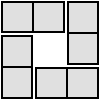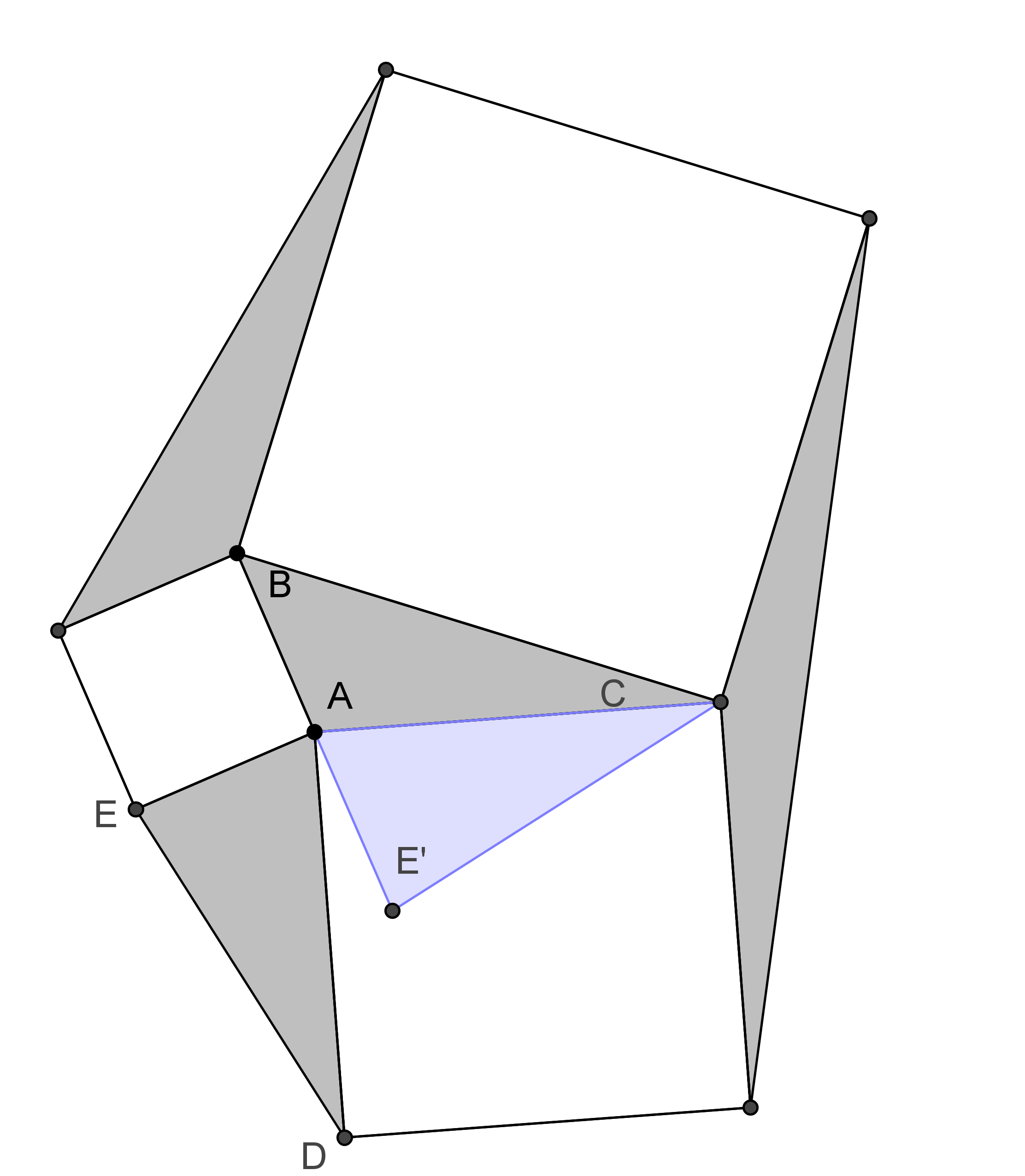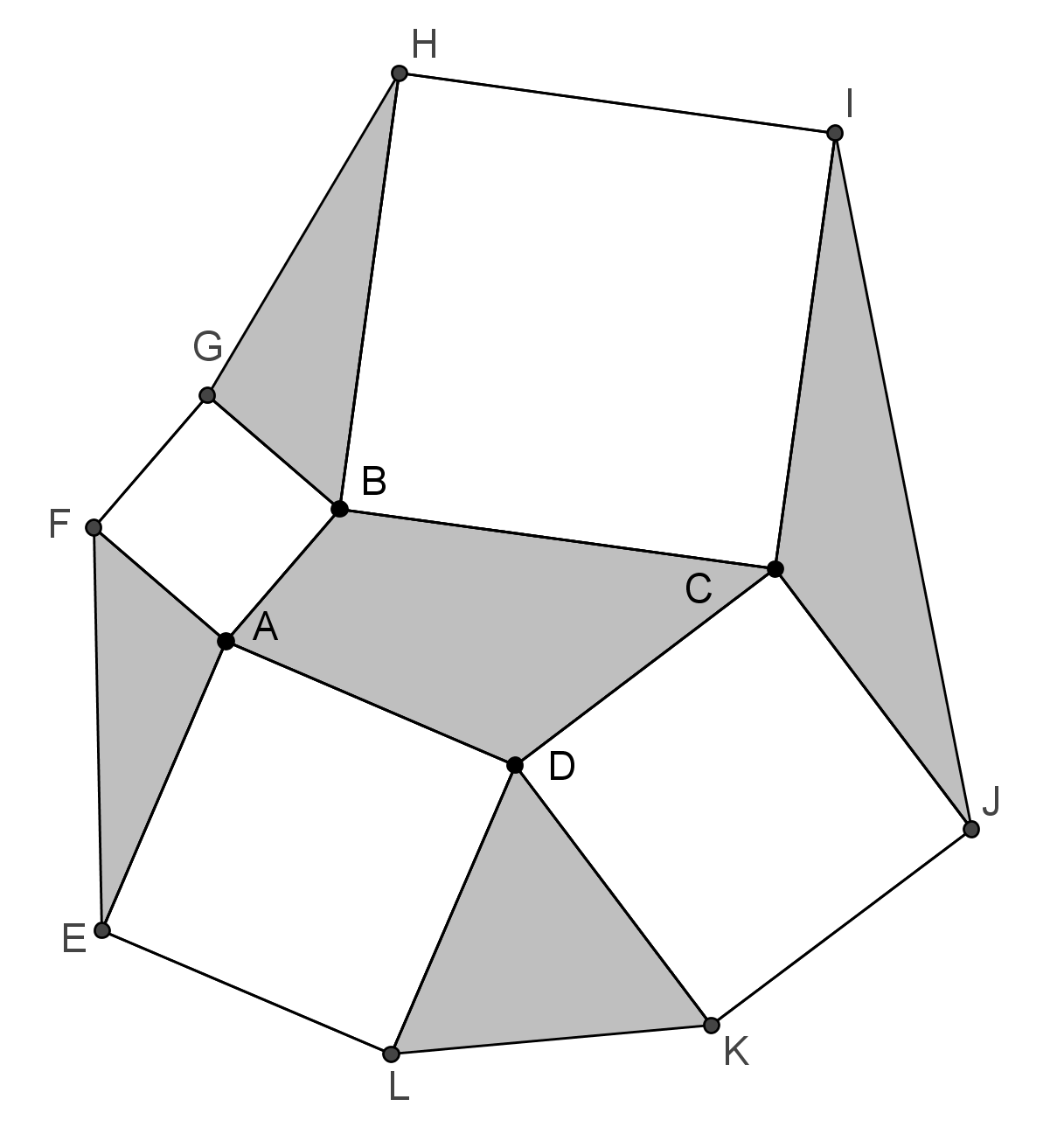#### You may also like### 4 Dom

Use these four dominoes to make a square that has the same number of dots on each side.### Just Rolling Round

P is a point on the circumference of a circle radius r which rolls, without slipping, inside a circle of radius 2r. What is the locus of P?### Areas and Ratios

Do you have enough information to work out the area of the shaded quadrilateral?

# Surprising Equalities

##### Age 14 to 18Challenge Level
For this problem, you might like to use dynamic geometry software such as GeoGebra, which is free to download and use. If you have never used GeoGebra before, you might like to read this article first.Draw any triangle $ABC$ and construct squares on each of its sides.

Join the vertices of the squares as shown in the diagram to create three more triangles.

Work out the area of the four triangles.
What do you notice?

Can you prove it?

Take a look at the Getting Started section to explore some interactive diagrams, or click below to see an image together with some questions which might help you to construct a proof.What transformation takes the point E to the point E'?

How does triangle ACE' relate to triangle ADE?

What proportion of triangle BCE' is triangle ABC?

Can you rotate the other grey triangles in a similar way?Here is a similar picture, which shows a quadrilateral $ABCD$ with a square constructed on each of its edges.

Triangles, which have been shaded, have been constructed between the squares.

Use your previous result to show that the areas of opposite triangles sum to the area of the quadrilateral $ABCD$.Finally, this picture is constructed around an unremarkable triangle, with two arbitrary squares constructed on one edge.

Single squares are then constructed on each of the remaining edges of the triangle.

Triangles are then drawn between the squares as shown.

Use your previous results to prove that the area of the darker shaded triangle $CIL$ is equal to the sum of the areas of the other two triangles $AKF$ and $BGJ$.